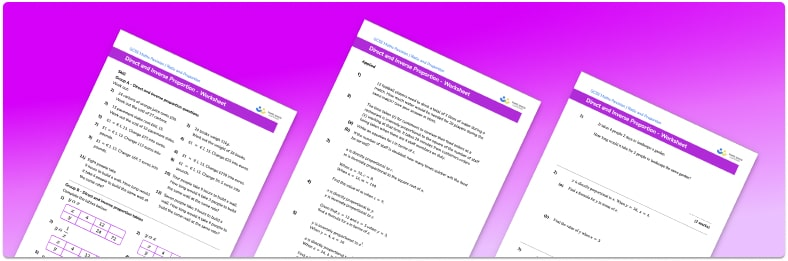# Direct and inverse proportion worksheet• Section 1 of the direct and inverse proportion worksheet contains 20+ skills-based direct and inverse proportion questions, in 3 groups to support differentiation
• Section 2 contains 3 applied direct and inverse proportion problems with a mix of worded problems and deeper problem solving questions
• Section 3 contains 3 foundation and higher level GCSE exam style questions on direct and inverse proportion
• Answers and a mark scheme for all direct and inverse proportion questions are provided
• Questions follow variation theory with plenty of opportunities for students to work independently at their own level
• All questions created by fully qualified expert secondary maths teachers

Suitable for GCSE maths revision for AQA, OCR and Edexcel exam boards

• This field is for validation purposes and should be left unchanged.

You can unsubscribe at any time (each email we send will contain an easy way to unsubscribe). To find out more about how we use your data, see our privacy policy.

### Direct and inverse proportion at a glance

Direct and inverse proportion are relationships between variables. In direct proportion, as one variable increases, the other variable increases at a linked rate, one variable is a multiple of the other. The constant of proportionality tells us how many times bigger one variable is than the other. Examples of directly proportional relationships include the amount someone is paid given the number of hours they work and the area of a circle given the square of its radius.

In inverse proportion, as one variable increases, the other decreases. Examples of this include the amount of time taken on a journey given the speed travelled or the amount of time taken to do a job given the number of people working on it.

Direct and inverse proportion is covered in both foundation and higher GCSE maths and in higher we look at an algebraic way to determine the constant of proportionality and solve proportion problems.

Looking forward, students can then progress to additional ratio and proportion worksheets, for example a ratio worksheet or a simplifying ratios worksheet.For more teaching and learning support on Ratio and Proportion our GCSE maths lessons provide step by step support for all GCSE maths concepts.

## Do you have KS4 students who need more focused attention to succeed at GCSE?There will be students in your class who require individual attention to help them succeed in their maths GCSEs. In a class of 30, it’s not always easy to provide.

Help your students feel confident with exam-style questions and the strategies they’ll need to answer them correctly with our dedicated GCSE maths revision programme.

Lessons are selected to provide support where each student needs it most, and specially-trained GCSE maths tutors adapt the pitch and pace of each lesson. This ensures a personalised revision programme that raises grades and boosts confidence.

Find out more